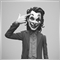# Integer division636

```int x = 3, y = 6;
double z;

z = y/x  ------  z = 2

z = x/y  ------ z = 0

z = (double)x/y ------ z = 0```

MQL4 documentation identifies this problem but the trouble is it doesn't say the correct way to get the right answer!!

Is the only way to get the right answer to declare x & y as 'double' even though in the program they are clearly integers?16412

sd59:

MQL4 documentation identifies this problem but the trouble is it doesn't say the correct way to get the right answer!!

Is the only way to get the right answer to declare x & y as 'double' even though in the program they are clearly integers?

`z = x/(double)y;`636

Doh! The simplest things.......Thanks!